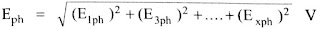# Effect of Harmonic Components on EMF of Synchronous Generator

0
357

### Effect of Harmonic Components on EMF of Synchronous Generator

#### These non-sinusoidal waveforms are expressed in terms of Fourier transforms which are the sum of series of sinusoidal waveforms.Now let us understand the effect of harmonic components on the synchronous generator.####Area of each fundamental pole,

A1 = πdl/P

ec = [Bm1 A1 2f sin θ + Bm3 A1 2f sin 3θ +……….. + Bmx A1 2f sin xθ]

Area of xth harmonic pole, Ax =  πdl/xP =  A1/x

#### ec = πf (Φ1  sin θ + 3Φ3 sin 3θ +……….. + xΦx  sin xθ)

Instantaneous value of fundamental frequency EMF generated in a conductor is,
ec1 = πfΦ1 sin θ    V

#### Hence the R.M.S. value of fundamental frequency EMF generated in a conductor is,

ec1 = πfΦ1/√2 = 2.22 fΦ1
Hence RMS value of xth harmonic frequency emf generated in a conductor is,#### The RMS value of resultant EMF of a conductor is,### Effect of Harmonic Components on Pitch Factor in Synchronous Generator:

#### We know that,

α = Angle of the short pitch for fundamental flux wave then it changes for the various harmonic component of flux as,

### Effect of Harmonic Components on Distribution Factor in Alternators:

#### The general expression to obtain distribution factor is,### Total EMF Generated due to Harmonics in synchronous Generator:

#### Consider the windings to short pitch and distributed, the e.m.f. of a fundamental  frequency is given by,

E1ph = 4.44 Kc1 Kd1 Φ1 f Tph   V

where               Tph = Turns per phase series
Φ1  = Fundamental flux component

While the phase e.m.f. of Xth order harmonic component of frequency is given by,

#### The total phase EMF is given by,#### Line EMF: For star connected, the line or terminal induced EMF is √3 times the total phase EMF.But it should be noted that with star connection, the 3rd harmonic voltages do not appear across line terminals though present in phase voltage.#### Taking the ratio of fundamental frequency EMF and Xth order harmonic frequency EMF we can write,#### From the ratio in above equation, we can write